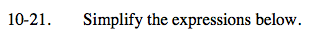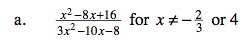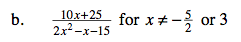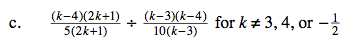### Home > CAAC > Chapter 10 > Lesson 10.1.2 > Problem10-21

10-21.Factor the numerator and denominator using a generic rectangle and the diamond pattern from earlier chapters.

$\frac{(x-4)(x-4)}{(x-4)(3x+2)}$

$\frac{(x-4)}{(3x+2)}$Follow the steps in part (a).Rewrite the quotient as a product.

$\frac{(k-4)(2k+1)}{5(2k+1)}\left(\frac{10(k-3)}{(k-3)(k-4)}\right)$

Simplify the expression and then look for factors that simplify to one.

$\frac{(k-4)(2k+1)(10)(k-3)}{5(2k+1)(k-3)(k-4)}$

$\frac{(k-4)}{(k-4)}=\frac{(2k+1)}{(2k+1)}=\frac{(k-3)}{(k-3)}=1$

$\frac{10}{5}=2$